# Analog Electronics - 7

## 10 Questions MCQ Test RRB JE for Electronics & Communication Engineering | Analog Electronics - 7

Description
Attempt Analog Electronics - 7 | 10 questions in 30 minutes | Mock test for Electronics and Communication Engineering (ECE) preparation | Free important questions MCQ to study RRB JE for Electronics & Communication Engineering for Electronics and Communication Engineering (ECE) Exam | Download free PDF with solutions
QUESTION: 1

### For the Wien-bridge oscillator circuit shown below, the condition for bridge balance is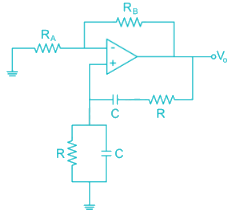Solution:

At oscillator frequency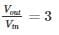i.e. gain of non-inverting amplifier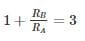⇒ RB = 2RA

QUESTION: 2

### Find the value of inductance in (mH) in the oscillator shown below to achieve a frequency of oscillation = 100 KHz is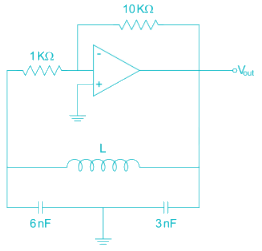Solution: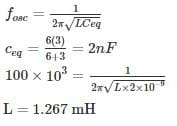*Answer can only contain numeric values
QUESTION: 3

### For the circuit shown in the figure the value of R to get oscillation of 100 kHz is ________ KΩ.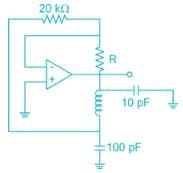Solution:

The given oscillator is Colpitts’s oscillator

The condition for oscillation is

|Aβ|=1

A = -R2/R1

β=C2/C1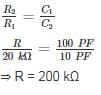*Answer can only contain numeric values
QUESTION: 4

The figure shows a phase-shift oscillator circuit with voltage follower buffer stages.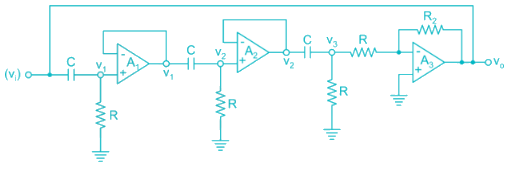The value of R2/R required for sustained oscillations is _____.

Solution:
• The basic amplifier of the circuit is the op-amp A3.
• The output of amplifier is connected to 3 stage RC filter.

Transfer function of first RC network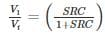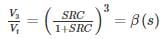The amplifier A3 is connected in inverting mode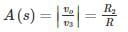The loop gain is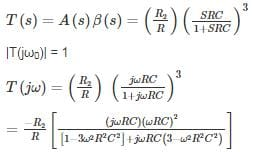Substitute real part of denominator to zero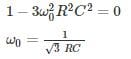At this frequency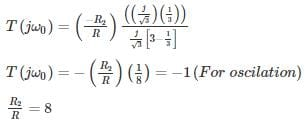QUESTION: 5

The value of R in oscillator circuit shown in a given circuit below is chosen such that it oscillates at an angular frequency of ω. The value of ω and the required value of R will respectively be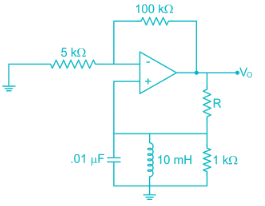Solution:

Hartley oscillator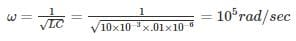Amp with +ve F.B

Loop again AB = 1 + j0

At oscillation

∠AB = 0 (no reactance XL = XC)

At frequency of oscillation look lies resistive Network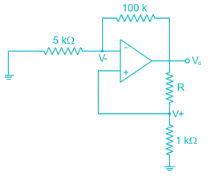For ideal op amp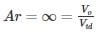Use voltage division Rule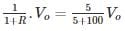R + 1 = 21

R = 20 kΩ

R = 2 × 104Ω

*Answer can only contain numeric values
QUESTION: 6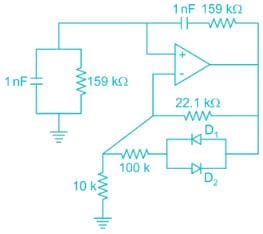The frequency of oscillation of the circuit in kilohertz is ______ kHz.

Solution:

The given circuit is wein bridge oscillator.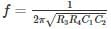R3 = R4 = 159 KΩ

C1 = C2 = 1 nF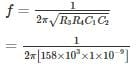= 103 HZ

= 1 KHZ

QUESTION: 7

For the transistor oscillator circuit given below the frequency of oscillation is 8.5 kHz. The value of the collector resistor approximately is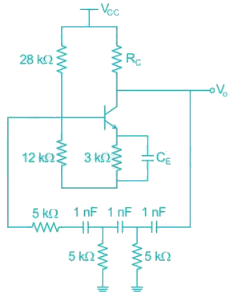Solution:

In a transistor phase shift oscillator the oscillation frequency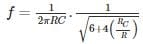R, C are the resistor and the capacitor in phase shift circuit

RC is collector capacitance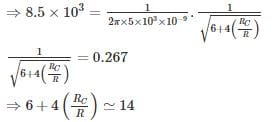⇒ RC = 2R = 10 kΩ

*Answer can only contain numeric values
QUESTION: 8

The oscillation frequency if the below-shown oscillator is 1 kHz then the value of ‘L’ is ______ mH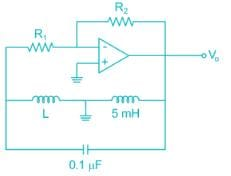Solution:

The given oscillator is Hartley oscillator, where the oscillation frequency.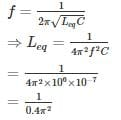= 253.3 mH

Leq = L + 5 mA

⇒ L = 253.3 – 5

= 248. 3 mH

QUESTION: 9

For the circuit shown the value of R1 and R2 such that the circuit generates oscillations of frequency 274 Hz is [Assume the time constant of the feedback RC network is 0.01 sec]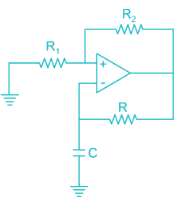Solution:

Given figure is of A-stable multi-vibrator,

Time constant is given by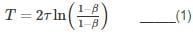β = feedback factor

τ = time constant of feedback loop: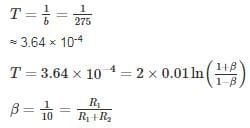Option 3 satisfies the condition

*Answer can only contain numeric values
QUESTION: 10

The frequency of oscillation of the circuit shown below is ________ Hz.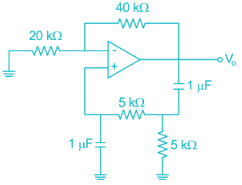Solution:

The given oscillator is Wien-bridge oscillator with R = 5 kΩ, C = 1 μF

⇒ frequency of oscillation f = 1/2πRC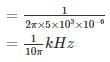= 31.8 HzUse Code STAYHOME200 and get INR 200 additional OFF Use Coupon Code